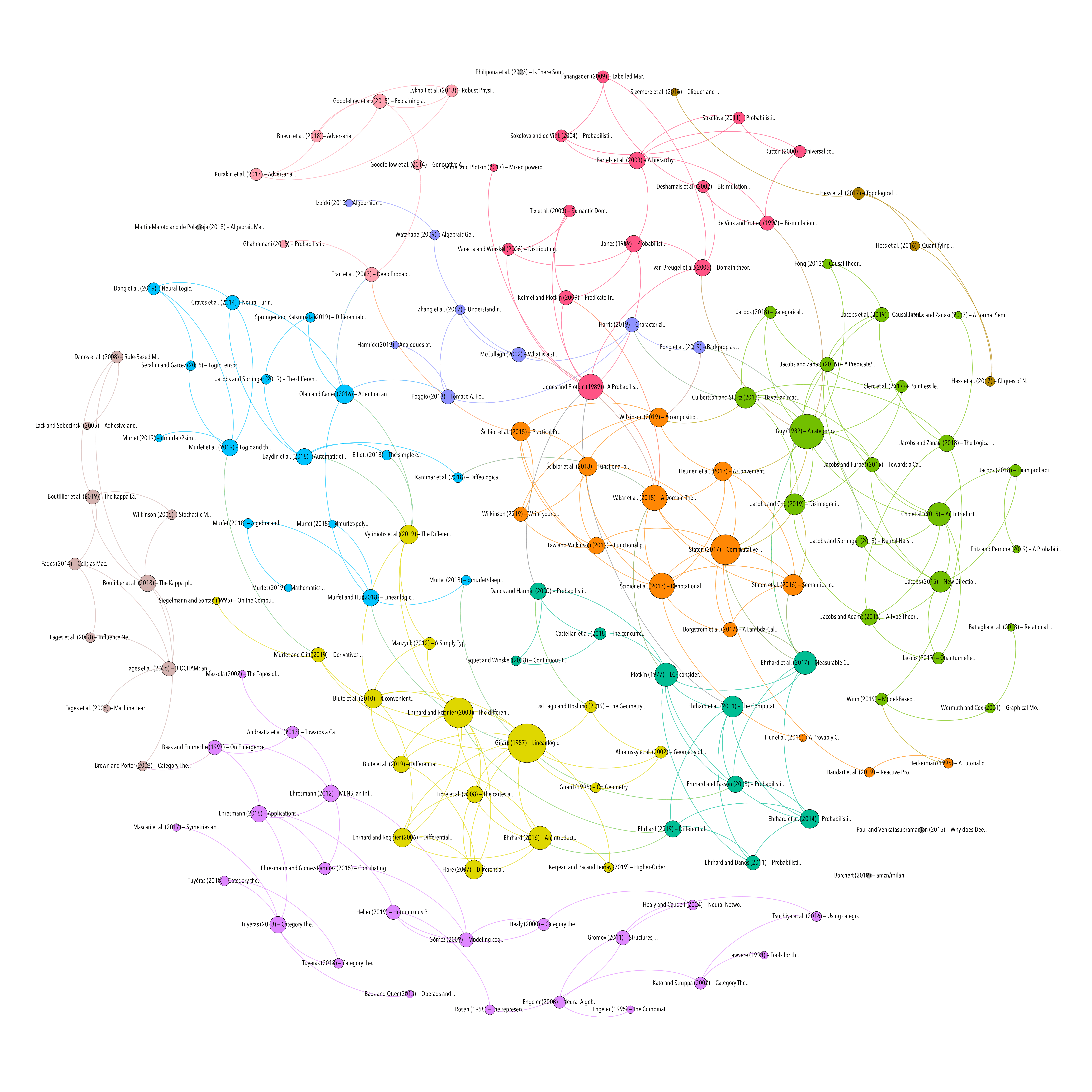Topic
Publication year

## An introduction to Differential Linear Logic: proof-nets, models and antiderivatives

Resource type
Author/contributor
Title
An introduction to Differential Linear Logic: proof-nets, models and antiderivatives
Abstract
Differential Linear Logic enriches Linear Logic with additional logical rules for the exponential connectives, dual to the usual rules of dereliction, weakening and contraction. We present a proof-net syntax for Differential Linear Logic and a categorical axiomatization of its denotational models. We also introduce a simple categorical condition on these models under which a general antiderivative operation becomes available. Last we briefly describe the model of sets and relations and give a more detailed account of the model of finiteness spaces and linear and continuous functions.
Publication
arXiv:1606.01642 [cs]
Date
2016-06-06
Short Title
An introduction to Differential Linear Logic
Accessed
2019-11-28T11:52:31Z
Library Catalog
Extra
ZSCC: 0000002 arXiv: 1606.01642
Citation
Ehrhard, T. (2016). An introduction to Differential Linear Logic: proof-nets, models and antiderivatives. ArXiv:1606.01642 [Cs]. Retrieved from http://arxiv.org/abs/1606.01642
CATEGORICAL LOGIC
DIFFERENTIAL CALCULUS
Topic
Attachment
Processing time: 0.03 secondsGraph of references (from Zotero to Gephi via Zotnet with this script)